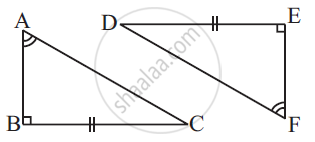Share

# Explain, Why Triangle Abc Congruence Triangle Fed. - CBSE Class 7 - Mathematics

ConceptCriteria for Congruence of Triangles

#### Question

Explain, why ΔABC ≅ ΔFED.#### Solution

Given that, ∠ABC = ∠FED (1)

∠BAC = ∠EFD (2)

The two angles of ΔABC are equal to the two respective angles of ΔFED. Also, the sum of all interior angles of a triangle is 180º. Therefore, third angle of both triangles will also be equal in measure.

∠BCA = ∠EDF (3)

Also, given that, BC = ED (4)

By using equation (1), (3), and (4), we obtain

ΔABC ≅ ΔFED (ASA criterion)

Is there an error in this question or solution?

#### APPEARS IN

NCERT Solution for Mathematics for Class 7 (2018 to Current)
Chapter 7: Congruence of Triangles
Ex. 7.20 | Q: 10 | Page no. 151
Solution Explain, Why Triangle Abc Congruence Triangle Fed. Concept: Criteria for Congruence of Triangles.
S